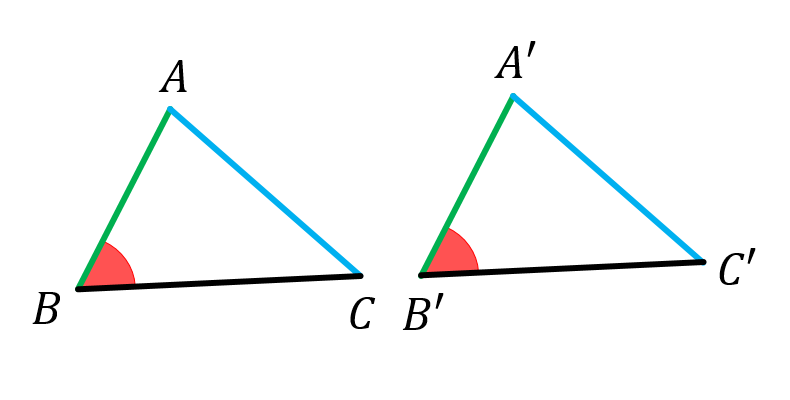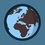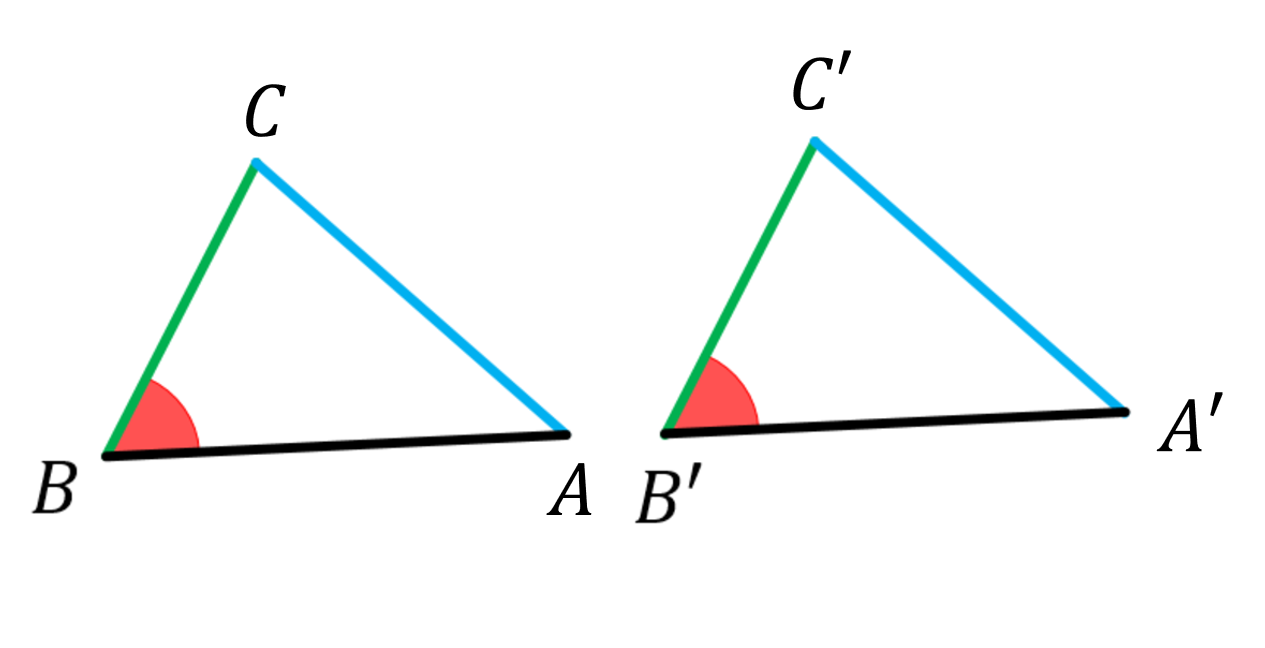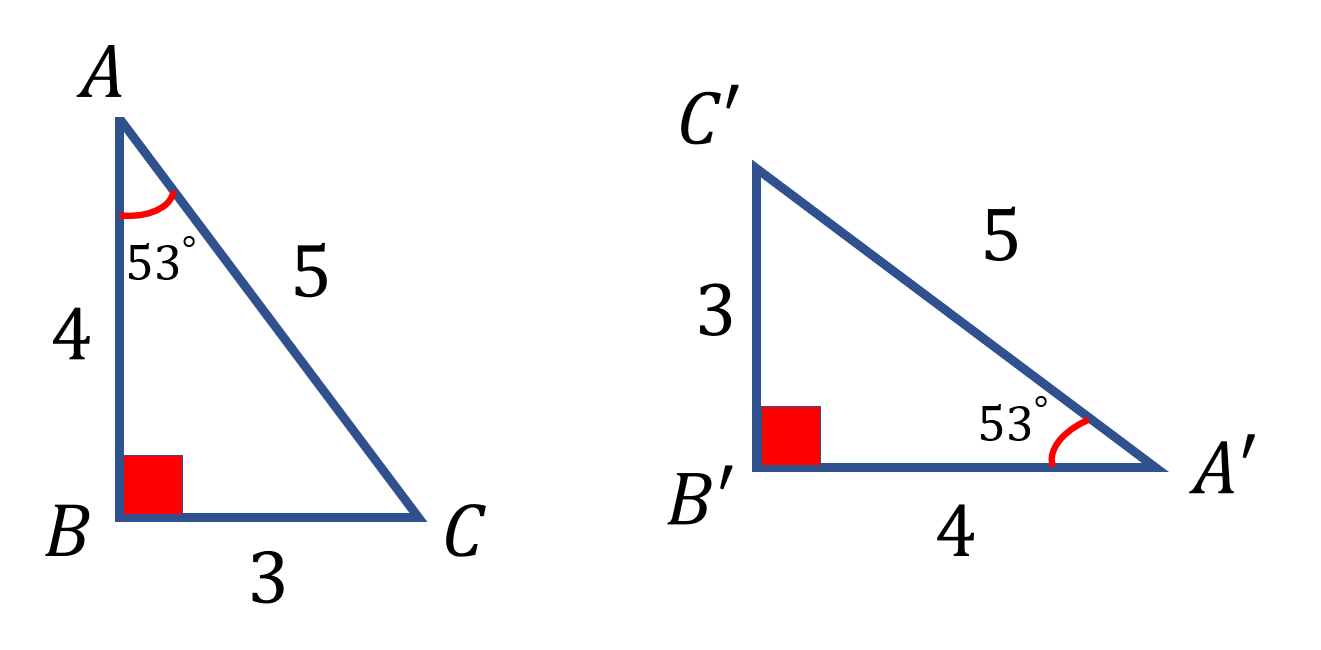# Identical triangles

• If two sides of two triangles are equal, and if one of their angles (faced?) to the non-smaller side are equal, then the triangles are identical.

So if $$AB=A'B';AC=A'C';AB\leq BC;\angle B=\angle B'$$#### I got it already. But you can write it if you wantNote by Páll Márton (no activity)
2 months, 1 week ago

This discussion board is a place to discuss our Daily Challenges and the math and science related to those challenges. Explanations are more than just a solution — they should explain the steps and thinking strategies that you used to obtain the solution. Comments should further the discussion of math and science.

When posting on Brilliant:

• Use the emojis to react to an explanation, whether you're congratulating a job well done , or just really confused .
• Ask specific questions about the challenge or the steps in somebody's explanation. Well-posed questions can add a lot to the discussion, but posting "I don't understand!" doesn't help anyone.
• Try to contribute something new to the discussion, whether it is an extension, generalization or other idea related to the challenge.

MarkdownAppears as
*italics* or _italics_ italics
**bold** or __bold__ bold
- bulleted- list
• bulleted
• list
1. numbered2. list
1. numbered
2. list
Note: you must add a full line of space before and after lists for them to show up correctly
paragraph 1paragraph 2

paragraph 1

paragraph 2

[example link](https://brilliant.org)example link
> This is a quote
This is a quote
    # I indented these lines
# 4 spaces, and now they show
# up as a code block.

print "hello world"
# I indented these lines
# 4 spaces, and now they show
# up as a code block.

print "hello world"
MathAppears as
Remember to wrap math in $$ ... $$ or $ ... $ to ensure proper formatting.
2 \times 3 $2 \times 3$
2^{34} $2^{34}$
a_{i-1} $a_{i-1}$
\frac{2}{3} $\frac{2}{3}$
\sqrt{2} $\sqrt{2}$
\sum_{i=1}^3 $\sum_{i=1}^3$
\sin \theta $\sin \theta$
\boxed{123} $\boxed{123}$

Sort by:

There are only five ways to prove congruency: SSS, SAS, ASA, AAS, and RHS. The SSA congruency theorem is not valid for all triangles. This might help. Sal shows why the length should be bigger.

- 2 months, 1 week ago

What is HL? Is it RHS? :)

- 2 months, 1 week ago

- 2 months, 1 week ago

My teacher explained us, so I know :)

- 2 months, 1 week ago

- 2 months, 1 week ago

Thank you! I got it. But if we know some other information, then it can be the smaller. Upvoted :)

- 2 months, 1 week ago

Its not necessary, the following two triangles are also congruent:- 2 months, 1 week ago

You should change the angles :)

- 2 months, 1 week ago

I don't understand. Wait, I am making another picture.

- 2 months, 1 week ago

Ok. I'm waiting. The marked angle should be A

- 2 months, 1 week ago

These 2 are also congruent:- 2 months, 1 week ago